## 4: The Graham Explained

As the escape wheel rotates, it exerts a force upon anything it encounters in its path. The direction of this force depends on what point on the escape wheel the pallet is located, as shown here: the arrows show the different directions of force for three points on the escape wheel.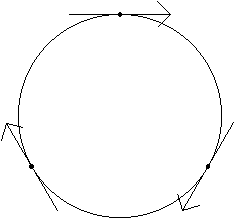If the top of the page were North, the escape wheel would push on the entry pallet towards the North-East. This force has size and direction. I have labeled it 'Fe1,' or the force exerted by the escape wheel. At this point, the pallet rotates clockwise: a force should push it in a North-West direction, marked 'Fp1' (force to pallet). The angle between Fe1 and Fp1 is 90º. If a third line were introduced, labeled 'Fi' (force of impulse), with an angle half way in between, you could think of an impulse force in that direction.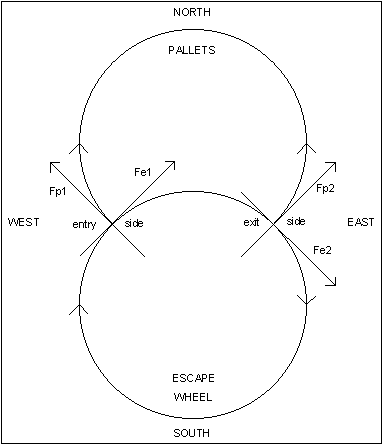We have Fi because a force cannot be rotated by 90º in the Graham escapement, which is why no power is transferred to the pallet from the escape wheel when the pallet's impulse face is at 90º or 0º to Fe. It could be rotated twice by 45º, though. Fi is at 90º to the impulse face's angle, so if the impulse face's angle were changed, Fi would change.

As Fe1 goes North-East, imagine that a portion of that force is received by the impulse face at right angles to its angle: Fi due North. Then imagine that a portion of Fi acts in a North-West direction, Fp1, to rotate the pallet. The portion of Fe1 not received in Fi is lost, and so is the portion of Fi not received in Fp1, which means that power is lost in each step.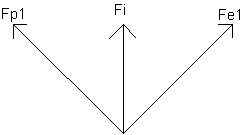If the lines were drawn to scale, you could see how much power is lost. Scale drawings could be used to show the effect of angle on Fi and Fp: changing the angle of the impulse face has a dramatic effect on the force Fp1 that rotates the pallet, even though the angle between Fe1 and Fp1 remains unchanged at 90º. If the angle FeFi were 25º, the efficiency would be 38%.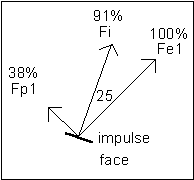If the angle were 45º, the efficiency would be 50%.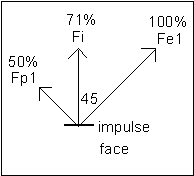If the angle were 65º, the efficiency would be 38%.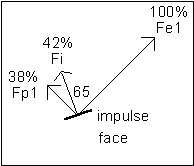The greatest efficiency is achieved when the angle of Fi is half way between those of Fe1 and Fp1.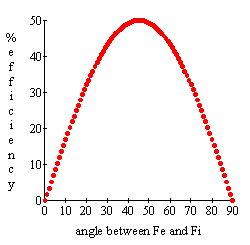On the exit side, Fe2 goes South-East and Fp2 goes North-East. Since Fi should go at an angle half way in between, Fi goes East.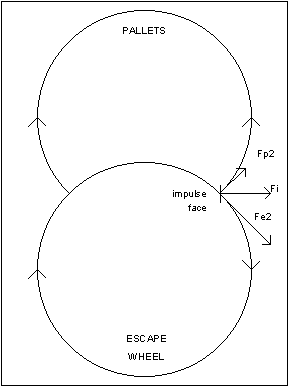An angle of 90º between Fe and Fp is preferred because of symmetry: the angle Fe1Fp1 plus the angle Fe2Fp2 always equals 180º. Therefore, if the angle Fe1Fp1 were greater than 90º, the angle Fe2Fp2 would be less than 90º to the same extent. Or vice versa.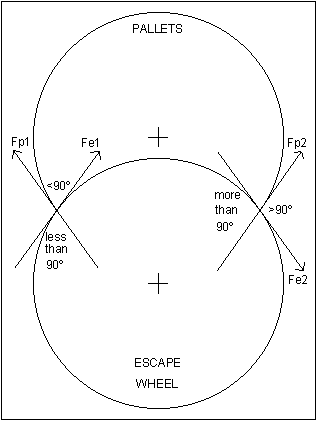As the angle FeFp increases, the maximum achievable efficiency decreases, so the smallest angle possible is preferred. The loss of efficiency on one side is equal to the gain in efficiency on the other side, but there is no advantage in an unequal arrangement. When the efficiencies are unequal, the push the pendulum receives on one side is different versus the other side, instead of the same. In the Graham escapement, therefore, the angle FeFp should be 90º because of symmetry. This is illustrated by the following drawing, which should be familiar to many clockmakers.

This approach could be used to determine the distance between the escape circle center and the pallets' circle center for the tooth span (the number of teeth between the pallets) of your choice: choose a 4.5 tooth span for a wide pendulum swing, or an 11.5 tooth span for a narrow swing. It is generally accepted that a 7.5 tooth span gives the most desirable results in practice for a 30 tooth escape wheel.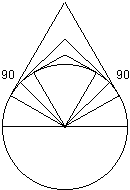Go to Chapter 5